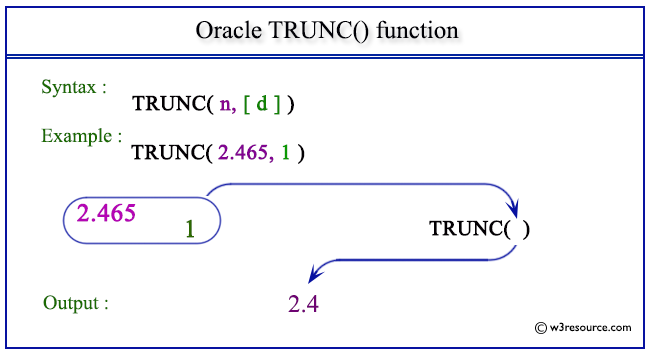# Oracle TRUNC() function

## Description

This function is used to truncate a number (n1) to a specified number of decimal places (n2).
When n2 is omitted, then n1 is truncated to 0 places.
n2 can be negative to truncate (make zero) n2 digits left of the decimal point.

Syntax:

```TRUNC(n1 [, n2 ])
```

Arguments:

Name Description
n1 A number which is to be truncated up to n2 decimal places.
n2 A number indicating up to how many decimal places, n1 is to be truncated.

Pictorial Presentation of TRUNC() functionExample:

``````SELECT TRUNC(2.465,1) FROM dual;
```
```

Here is the result.

```TRUNC(2.465,1)
--------------
2.4
```

The above statement will return a value truncating 2.465 up to 1 decimal place.

Example: TRUNC() function with negative decimal places

``````SELECT TRUNC(142.465,-2) FROM dual;
```
```

Here is the result.

```TRUNC(142.465,-2)
-----------------
100
```

The above statement will return a value truncating 142.465 up to -2 decimal places.

Previous: TANH
Next: WIDTH_BUCKET

﻿